# An iterative pilot-data-aided estimator for SFBC relay-assisted OFDM-based systems

## Abstract

In this article, we propose and assess an iterative pilot-data-aided channel estimation scheme for space frequency block coding relay-assisted OFDM-based systems. The relay node (RN) employs the equalise-and-forward protocol, and both the base station (BS) and the RN are equipped with antenna arrays, whereas the user terminal (UT) is a single-antenna device. The channel estimation method uses the information carried by pilots and data to improve the estimate of the equivalent channels for the path BS-RN-UT. The mean minimum square error criterion is used in the design of the estimator for both the pilot-based and data-aided iterations. In different scenarios, with only one data iteration, the results show that the proposed scheme requires only half of the pilot density to achieve the same performance of non-data-aided schemes.

## 1. Introduction

Multiple-input and multiple-output (MIMO)-based schemes exploit the benefits from the spatial diversity to enhance the link reliability and achieve high throughput. In some situations however, the integration of multiple antenna elements is unpractical especially in mobile terminals due to the size and power constraints. In order to overcome this shortcoming, virtual antenna-array has emerged as a solution to obtain spatial diversity in a distributed approach. The use of dedicated equipment with relaying capabilities rose as a promising technique to expanded coverage, system wide power savings and better immunity against signal fading . The cooperation is enabled by a relaying protocol , e.g., decode-and-forward (DF) when the relay has the capability to regenerate and re-encode the whole frame; amplify-and-forward (AF) where only amplification takes place; and what is designated as equalise-and-forward (EF) [3, 4], where more sophisticated filtering operations are used.

A large number of cooperative techniques have been reported in the literature showing the potential of relay-assisted scenarios. In order to exploit the full potential of cooperative communication, accurate estimates for the different links are required. Although some work has evaluated the impact of the imperfect channel estimation in cooperative schemes , new techniques have also been proposed that address the specificities of such systems. While with the DF protocol, channel estimation algorithms developed for point-to-point links can be used without modifications, the situation is different when employing AF or techniques performing linear filtering at the relay node (RN). In the later, the overall channel from the base station (BS) to the user terminal (UT) is a composite one with an additional source of noise degrading the performance of point-to-point techniques .

This has motivated research on channel estimation considering AF and different scenarios . In , the overall channels are estimated at the UT through classical estimators based on a pre-defined amplifying matrix at the RN. The authors of  proposed a matrix-based algorithm for channel estimation considering an optimisation problem based on the normalised least mean square (NLMS) cost function. In the same way, the authors of  used a similar optimisation problem considering the recursive NLMS. The use of complex polyphase sequences to estimate the channel impulse response (CIR) of the equivalent channel was proposed in . In , the authors presented a tensor-based channel estimation algorithm with an iterative scheme based on the structured least square to refine the initial estimation. Transceiver schemes that jointly design the relay forward matrix and the destination equaliser that minimise the MSE have been proposed in . Concerning the two-way relay, the authors of  proposed an estimator based on new training strategy to jointly estimate the channels and frequency offset. For MIMO relay channels, the linear mean square error (MSE) estimator and optimal training sequences to minimise the MSE are derived in .

The estimation methods of the previously referred work were based on pilots or training sequences. However, the channels present in a cooperative scenario can also be estimated or aided using the energy of the transmitted data . In , a recursive channel estimation method based on the channel coder feedback information and linear interpolation is proposed. In  is presented an estimator method that obtain initial estimation based on maximum likelihood and improve it via expectation and maximisation (EM). In , the authors proposed an iterative channel estimator based on the EM algorithm to separately estimate the channels B → R (BS-RN) and R → U (RN-UT), that on the initial phase uses a training sequence and after can use the regenerated data. Although not using directly the regenerated data, in  superposition of pilots and data was considered and based on the non-Gaussian nature of the dual-hop relay link, the authors proposed a first-order autoregressive channel model and derived a Kalman filter-based estimator.

The works discussed above consider only single-antenna network elements (source, relay and destination). However, in several scenarios, namely in the downlink of cellular systems, it is both feasible and beneficial to consider the BS and the RN (if dedicated) with antenna arrays allowing space diversity [3, 4]. In these cases, there is a need for more complex equalisation at the relay since the use of AF limits the exploitation of space diversity provided by the use of multiple antennas. With this scheme, that we term EF, we obtain similarly to the AF protocol, an equivalent channel with additional sources of distortion that requires improved channel estimation schemes. Unlike the AF case, for which several proposals have been published as we pointed out previously, channel estimation schemes that consider the composite channel of EF have not been reported in the literature. This manuscript address this problem and proposes a channel estimation scheme for the space frequency block coding (SFBC) relay-assisted scenario discussed in , where both BS and RN are equipped with an antenna array. This manuscript extends the work in [24, 25] by providing detailed derivations, considering additional scenarios and, unlike , using the information of the regenerated data to improve the channel estimates. The estimation method at the UT consists of two iterations; in the first one, only pilots are used to estimate the channels and the results are used to perform a first decision on the data symbol. Then, in the next iteration, these symbols are used as virtual pilots to improve the channel estimates to be used in the final symbol decision. The MMSE criterion is used in the design of the estimator for both the pilot-based and data-aided iterations. The results are compared against the pilot-based estimation scheme presented in  and they show that, for the same pilot density, the MSE reduces or, alternatively, fewer pilots are needed to achieve the same performance. Therefore, the system's spectral efficiency is improved with only one data iteration.

The remainder of this article is organised as follows. We present in Section 2 the system model and the mathematical description involving the cooperative transmission. In Section 3, we present the proposed estimator scheme. The results in terms of normalised MSE are presented in Section 4. Finally, the main conclusions are outlined in Section 5.

## 2. System model

The indicesand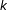denote time and frequency domain variables, respectively. $E\left\{\cdot \right\}$ is the statistical expectation operator, $\left(\circ \right)$, ${\left(\cdot \right)}^{T}$ and ${\left(\cdot \right)}^{*}$ are the pointwise, transpose and conjugate operations, respectively. $\mathsf{\text{diag}}\left(\cdot \right)$ stands for a diagonal matrix and $\mathsf{\text{FT}}\left(\cdot \right)$ denotes the Fourier transform operation. Variables, vectors or matrices in time domain (TD) are denoted by $\left(\stackrel{̃}{}\right)$. All estimates are denoted by $\left(\stackrel{^}{}\right)$.

### 2.1. Channel model

We consider an OFDM-based system with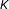subcarriers and time-variant channels with discrete impulse response of the type

${\stackrel{̃}{h}}_{\left(n\right)}=\sum _{g=1}^{G}{\beta }_{g\left(n\right)}\delta \left(n-{\tau }_{g}\right),$
(1)

whereis the instant when the CIR is evaluated,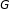is total number of paths, ${\beta }_{g}$ and ${\tau }_{g}$ are the complex amplitude and delay of the path. ${\beta }_{g}$ is modelled as a zero mean complex Gaussian variable with variance ${\sigma }_{g}^{2}$ determined by the power delay profile and satisfying ${\sum }_{g=1}^{G}{\sigma }_{g}^{2}=1$. Although the channel is time-variant we assume it quasi-static, i.e. constant during one OFDM symbol interval. In the frequency domain, the channel gains, ${h}_{\left(k\right)},k=0,\dots ,K-1$, are therefore also zero mean complex Gaussian variables with unit variance. It is widely known that in typical OFDM systems the subcarrier separation is significantly lower than the coherence bandwidth of the channel. Accordingly, the fading in two adjacent subcarriers can be considered flat and without loss of generality we can assume for generic channel ${h}_{\left(k\right)}={h}_{\left(k+1\right)}$. We also assume $E\left\{{\left|{h}_{\left(k\right)}\right|}^{2}\right\}=1$.

### 2.2. Relay-assisted (RA)/cooperative scheme

In this section, we briefly describe the downlink SFBC relay-assisted scenario considered since a detailed description can be found in . The scenario is depicted in Figure 1, where the BS transmits information to the UT using both the direct link and a dedicated relay.

The BS and the RN are equipped with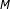andantennas, respectively. These scenarios are referred as $M×L×1$ schemes. The signals at the transmitter equipped with two antennas are SFBC encoded according to the Table 1. In the following, the indicesand, where $m=1,2$ and $l=1,2$, are related to the antennas at the BS and the RN, respectively. Therefore, the channels B → U, B → R and R → U are represented by ${}_{h}^{\mathsf{\text{bu}}m,\left(k\right)}$, ${}_{h}^{\mathsf{\text{br}}ml,\left(k\right)}$ and ${}_{h}^{\mathsf{\text{ru}}l,\left(k\right)}$, respectively.

We assume a half-duplex EF relaying protocol which requires two phases. In phase I, the encoded data $d$, with unit variance, are transmitted through the direct link to the UT and the link B → R to the RN. At the RN, linear operations that perform the Alamouti decoding and re-encode the soft estimates using the same scheme are performed. It should be emphasised that when the RN is equipped with an antenna array the AF protocol is not the best strategy  since it would not allow getting benefits of the space diversity provided by the use of multiple antennas. In such case, and assuming Rayleigh fading, for each data symbol the equivalent channel from source to one antenna element of the relay is the sum of two complex Gaussian random variables. Therefore, a $2×2×1$ system asymptotically achieves the same diversity as a $2×1×1$. Consequently, we need to perform an equalisation to decode and combine the received signals on each antenna before Alamouti re-encoding at the RN. However, in the considered protocol, no hard decision is performed at the RN, this fact being the reason to refer it as EF.

In phase II, while the BS is idle, the RN forwards the re-encoded signal. Therefore, the received signal at the UT per subcarriersand $k+1$ are given by

$\left\{\begin{array}{c}{}_{y}^{\mathsf{\text{ru}},\left(k\right)}=\frac{1}{\sqrt{2}}\left({h}_{\mathsf{\text{ru}}1,\left(k\right)}{\alpha }_{\left(k\right)}{}_{s}^{\mathsf{\text{br}},\left(k\right)}-{h}_{\mathsf{\text{ru}}2,\left(k+1\right)}{\alpha }_{\left(k+1\right)}^{*}{s}_{\mathsf{\text{br}},\left(k+1\right)}^{*}\right)+{}_{n}^{\mathsf{\text{ru}},\left(k\right)}\\ {}_{y}^{\mathsf{\text{ru}},\left(k+1\right)}=\frac{1}{\sqrt{2}}\left({h}_{\mathsf{\text{ru2}},\left(k\right)}{\alpha }_{\left(k\right)}^{*}{s}_{\mathsf{\text{br}},\left(k\right)}^{*}+{h}_{\mathsf{\text{ru1}},\left(k+1\right)}{\alpha }_{\left(k+1\right)}{}_{s}^{\mathsf{\text{br}},\left(k+1\right)}\right)+{}_{n}^{\mathsf{\text{ru}},\left(k+1\right)}\end{array}\right\,$
(2)

where ${}_{n}^{\mathsf{\text{ru}},\left(k\right)}$ is the additive Gaussian noise with zero mean and variance ${\sigma }_{\mathsf{\text{ru}}}^{2}$ and ${\alpha }_{\left(k\right)}$ is a constant that constrains the overall power at the RN to one expressed by

${\alpha }_{\left(k\right)}={\left(\sqrt{{\mathrm{\Gamma }}_{\left(k\right)}^{2}+{}_{\mathrm{\Gamma }}^{\left(k\right)}{\sigma }_{\mathsf{\text{br}}}^{2}}\right)}^{-1}.$
(3)

${s}_{\mathsf{\text{br}},\left(k\right)}$ is the soft estimate of the SFBC de-mapping, given by

$\left\{\begin{array}{l}{}_{s}^{\mathsf{\text{br}},\left(k\right)}={\mathrm{\Gamma }}_{\left(k\right)}{d}_{\left(k\right)}+{}_{q}^{\mathsf{\text{br,}}\left(k\right)}\\ {}_{s}^{\mathsf{\text{br}},\left(k+1\right)}={\mathrm{\Gamma }}_{\left(k\right)}{d}_{\left(k+1\right)}+{}_{q}^{\mathsf{\text{br,}}\left(k+1\right)}\end{array}\right\,\phantom{\rule{1em}{0ex}}{}_{\mathrm{\Gamma }}^{\left(k\right)}=\frac{1}{2}\sum _{m=1}^{2}\sum _{l=1}^{2}{\left|{ĥ}_{\mathsf{\text{br}}ml,\left(k\right)}\right|}^{2},$
(4)

${q}_{\mathsf{\text{br}},\left(k\right)}$ representing the noise term that is transmitted by the RN.

Using the previous expressions, we can verify that the data component at the UT, received via the cooperative link is ${\alpha }_{\left(k\right)}{\mathrm{\Gamma }}_{\left(k\right)}{h}_{\mathsf{\text{ru}}l,\left(k\right)}{d}_{\left(k\right)}$ and therefore we can define the equivalent channel from the BS to UT, ${h}_{\mathsf{\text{eq}}l\mathsf{\text{,}}\left(k\right)}={\alpha }_{\left(k\right)}{\mathrm{\Gamma }}_{\left(k\right)}{h}_{\mathsf{\text{ru}}l,\left(k\right)}$. The SFBC de-mapping of the received signals at the UT, by the cooperative link, are given by

$\left\{\begin{array}{l}{}_{s}^{\mathsf{\text{ru}},\left(k\right)}={g}_{\mathsf{\text{ru}}1}^{*}{}_{y}^{\mathsf{\text{ru}},\left(k\right)}+{}_{g}^{\mathsf{\text{ru}}2}{y}_{\mathsf{\text{ru}},\left(k+1\right)}^{*},{g}_{\mathsf{\text{ru}}1}={h}_{\mathsf{\text{eq1,}}\left(k\right)}/\left(\sqrt{2}{\sigma }_{\mathsf{\text{t}}}^{2}\right)\\ {}_{s}^{\mathsf{\text{ru}},\left(k+1\right)}=-{}_{g}^{\mathsf{\text{ru}}2}{y}_{\mathsf{\text{ru}},\left(k\right)}^{*}+{g}_{\mathsf{\text{ru}}1}^{*}{}_{y}^{\mathsf{\text{ru}},\left(k+1\right)},{g}_{\mathsf{\text{ru}}2}={h}_{\mathsf{\text{eq2,}}\left(k\right)}/\left(\sqrt{2}{\sigma }_{\mathsf{\text{t}}}^{2}\right)\end{array}\right\.$
(5)

In (5), ${\sigma }_{\mathsf{\text{t}}}^{2}$ is the variance of the total noise given by

${\sigma }_{\mathsf{\text{t}}}^{2}={\alpha }_{\left(k\right)}^{2}{}_{\mathrm{\Gamma }}^{\left(k\right)}{}_{\mathrm{\Gamma }}^{\mathsf{\text{ru}},\left(k\right)}{\sigma }_{\mathsf{\text{br}}}^{2}+{\sigma }_{\mathsf{\text{ru}}}^{2},\phantom{\rule{1em}{0ex}}{}_{\mathrm{\Gamma }}^{\mathsf{\text{ru}},\left(k\right)}=\frac{1}{2}\sum _{l=1}^{2}{\left|{ĥ}_{\mathsf{\text{ru}}l,\left(k\right)}\right|}^{2},$
(6)

with ${\sigma }_{\mathsf{\text{br}}}^{2}$ being the variance of the total noise at the input of the RN.

The data symbols are obtained after performing the joint processing which corresponds to combining the soft-decision variables received in both phases of the protocol, i.e. via the direct and the cooperative links.

In order to estimate the channels, we consider the use of pilot symbols. At the BS, pilots are assumed to be constant during one OFDM symbol transmission. Data and pilot subcarriers are multiplexed according to the pilot pattern in Figure 2. Due to the fact that in this study both BS and RN are equipped with two antennas, we consider that each antenna path has different subsets of pilot subcarriers, according to Figure 2. Two consecutives pilot subcarriers are spaced by ${N}_{f}$, or $2{N}_{f}$ if considering a specific antenna.

At the BS, the pilots are considered unitary in all positions, i.e. $p=1$. At the RN, the same pilot positions are filled. According to Equations (4) and (5), in order to perform optimal equalisation, we need to estimate ${h}_{\mathsf{\text{eq}}l\mathsf{\text{,}}\left(k\right)}={\alpha }_{\left(k\right)}{\mathrm{\Gamma }}_{\left(k\right)}{h}_{\mathsf{\text{ru}}l,\left(k\right)}$ at the receiver and using $p=1$ will no longer provide the required channels estimates. Therefore, at the RN the pilot positions are filled with ${p}_{\left(k\right)}={\alpha }_{\left(k\right)}{\mathrm{\Gamma }}_{\left(k\right)}$.

## 3. Proposed pilot-data-aided estimator

The iterative pilot-data-based estimator presented in this study focuses on phase II where the channel estimator estimates only the relay/cooperative channels. The estimation processing follows Figure 3.

The superscriptindicates in which iteration $\left(i=1,2\right)$ the estimate is obtained. ${\stackrel{^}{D}}^{\left(1\right)}$ are the binary decoded data, ${\stackrel{^}{d}}^{\left(1\right)}$ represents the data symbols that are obtained after the re-modulation and ${ĥ}_{\mathsf{\text{ru}}l}^{\left(i\right)}$ corresponds to the channels estimates. The channels estimates ${ĥ}_{\mathsf{\text{ru}}l}^{\left(1\right)}$ are obtained using only pilot information, whereas for ${ĥ}_{\mathsf{\text{ru}}l}^{\left(2\right)}$ the data regenerated in iteration 1 is used to improve the estimates. In the second iteration, the pilot-data-based estimates ${ĥ}_{\mathsf{\text{ru}}l}^{\left(2\right)}$ are used to perform the SFBC de-mapping and the output is then fed to the Joint Processing block to produce the final data estimates.

### 3.1. Pilot-based estimation

#### 3.1.1. The TD-MMSE estimator

The initial estimation is obtained via pilots and it is accomplished according to the pilot-based Time Domain Mean Minimum Square Error (TD-MMSE) estimator . This method performs in TD the optimal estimation, i.e. the LS estimation and MMSE filtering. The operation in time domain leads to a significant complexity reduction relatively to the conventional frequency domain processing because the MMSE filter corresponds to a sparse diagonal matrix, as was extensively discussed in .

For one OFDM symbol withsubcarriers, two consecutive pilot subcarriers are spaced by ${N}_{f}$. According to the Nyquist theorem, summing ${N}_{f}$ delayed (by $K/{N}_{f}$) replicas of the input signal is equivalent to filter the pilot positions in the frequency domain, and therefore, the LS estimate in time-domain is made-up of ${N}_{f}$ replicas of the CIR separated by $K/{N}_{f}$

(7)

where $\stackrel{̃}{w}$ is the noise with noise variance ${\sigma }_{n}^{2}$.

For one OFDM symbol, the LS estimate is a vector $1×K$ where assuming the Nyquist criterion about pilot separation is fulfilled, the last $K-K/{N}_{f}$ elements are null

(8)

The LS estimate given by (8) is improved by using the MMSE filter that is implemented by $\left(K/{N}_{f}\right)×\left(K/{N}_{f}\right)$ matrices. For a generic channel, the TD MMSE filter is expressed by ${\mathbf{W}}_{\mathsf{\text{MMSE}},\stackrel{̃}{h}}={\mathbf{R}}_{\stackrel{̃}{h}\stackrel{^}{\stackrel{̃}{h}}}{\mathbf{R}}_{\stackrel{^}{\stackrel{̃}{h}}\stackrel{^}{\stackrel{̃}{h}}}^{-1}$, where ${\mathbf{R}}_{\stackrel{^}{\stackrel{̃}{h}}\stackrel{^}{\stackrel{̃}{h}}}$ is the filter input correlation, $\mathsf{\text{E}}\left\{\stackrel{^}{\stackrel{̃}{\mathbf{h}}}{\stackrel{^}{\stackrel{̃}{\mathbf{h}}}}^{H}\right\}$, which is given by ${\mathbf{R}}_{\stackrel{̃}{h}\stackrel{̃}{h}}+{\sigma }_{n}^{2}{\mathbf{I}}_{K/{N}_{f}}$ and ${\mathbf{R}}_{\stackrel{̃}{h}\stackrel{^}{\stackrel{̃}{h}}}$ is the filter input-output cross-correlation matrix $\mathsf{\text{E}}\left\{\stackrel{̃}{\mathbf{h}}{\stackrel{^}{\stackrel{̃}{\mathbf{h}}}}^{H}\right\}$, which is given by . If the channel taps are separated by the sampling interval, the MMSE filter in TD corresponds to a sparse $K/{N}_{f}$ diagonal matrix with non-null elements whose number is equal to the number of tapsoccurring only in the diagonal:

${\stackrel{̃}{\mathbf{W}}}_{\mathsf{\text{MMSE}}}=\mathsf{\text{diag}}\left(\frac{{\sigma }_{1}^{2}}{{\sigma }_{1}^{2}+\frac{{\sigma }_{n}^{2}}{K/{N}_{f}}},\dots ,\frac{{\sigma }_{G}^{2}}{{\sigma }_{G}^{2}+\frac{{\sigma }_{n}^{2}}{K/{N}_{f}}},0,\dots ,0\right).$
(9)

The two previous equations may be simultaneously implemented in order to minimise the estimator complexity, thus the final CIR estimate presentsnon-null elements and zeros in the remaining .

Therefore, atsubcarrier the element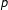of the pilot vector $\mathbf{p}$ may be expressed as a pulse train equispaced by ${N}_{f}$ with unitary amplitude. The corresponding expression in TD is also given by a pulse train with elements in the instants $\left(n-mK/{N}_{f}\right)$ for $m\in \left\{0,\dots ,{N}_{f}-1\right\}$, according to the following expression.

(10)

The transmitted signal is made-up of data and pilot components. Consequently, at the receiver side the component of the received signal in TD is given by

${ỹ}_{\left(n\right)}=\sum _{k=0}^{{N}_{c}-1}{\stackrel{̃}{h}}_{\left(k\right)}{d}_{\left(n-k\right)}+\frac{1}{{N}_{f}}\sum _{m=0}^{{N}_{f}-1}{\stackrel{̃}{h}}_{\left(n-mK/{N}_{f}\right)}+{ñ}_{\left(n\right)},$
(11)

where ${ñ}_{\left(n\right)}$ corresponds to the complex white Gaussian noise.

Convolving the expression in (11) with the pilots symbols ${\stackrel{̃}{p}}_{\left(n\right)}$ we obtain the expression in (7). This convolution corresponds to multiply the subcarriers at frequency ${N}_{f}$ by 1. By design, these are the positions reserved to the pilots thus the data component vanishes.

### 3.2. TD-MMSE estimator for the equivalent channel

According to the scenario presented in Section 2.2, we need to estimate the equivalent channel ${h}_{\mathsf{\text{eq}}l,\left(k\right)}={\alpha }_{\left(k\right)}{\mathrm{\Gamma }}_{\left(k\right)}{h}_{\mathsf{\text{ru}}l,\left(k\right)}$ that depends on ${\alpha }_{\left(k\right)}$ and ${\mathrm{\Gamma }}_{\left(k\right)}$. The UT is not aware of ${\alpha }_{\left(k\right)}{\mathrm{\Gamma }}_{\left(k\right)}$ since it is dependent on ${h}_{\mathsf{\text{br}}ml,\left(k\right)}$ and the UT is not aware of these channels as well. Nevertheless, the channels ${h}_{\mathsf{\text{br}}ml,\left(k\right)}$ are estimated at the RN, and based on that, ${\alpha }_{\left(k\right)}{\mathrm{\Gamma }}_{\left(k\right)}$ is computed and inserted in the pilot position as explained in Section 2.2. Since the new pilots ${\alpha }_{\left(k\right)}{\mathrm{\Gamma }}_{\left(k\right)}$ are not unitary, the convolution with the received signal results in overlapped replicas of the CIR, as shown in Figure 4. Therefore, it is important to assess the impact of using ${\alpha }_{\left(k\right)}{\mathrm{\Gamma }}_{\left(k\right)}$ as pilots on the estimator performance.

In Figure 5, we present the behaviour of ${\alpha }_{\left(k\right)}{\mathrm{\Gamma }}_{\left(k\right)}$ in terms of amplitude per subcarrier. We considered two values of ${E}_{\mathsf{\text{b}}}/{N}_{0}$, 2 and 20 dB, where Eb corresponds to the energy per bit received at UT and ${N}_{0}/2$ is the bilateral power spectrum density of the noise that affects the information conveying signals in a point-to-point link. For these results, we consider the channels according to ITU pedestrian, models A and B . According to the results for the ${\alpha }_{\left(k\right)}{\mathrm{\Gamma }}_{\left(k\right)}$ presents amplitude values close to 1 with some negligible fluctuation. However, for the result is slightly different to the previous one: ${\alpha }_{\left(k\right)}{\mathrm{\Gamma }}_{\left(k\right)}$ presents an amplitude also close to 1 but the fluctuation is not negligible.

This can easily be explained according to (3), ${\alpha }_{\left(k\right)}$ depends on the noise variance ${\sigma }_{\mathsf{\text{br}}}^{2}$ and therefore ${\alpha }_{\left(k\right)}{\mathrm{\Gamma }}_{\left(k\right)}$ tends to one for a high signal-to-noise (SNR) value, according to the following expression

${\alpha }_{\left(k\right)}{\mathrm{\Gamma }}_{\left(k\right)}=\left(\frac{1}{\sqrt{{\mathrm{\Gamma }}_{\left(k\right)}^{2}+{\mathrm{\Gamma }}_{\left(k\right)}{\sigma }_{\mathsf{\text{br}}}^{2}}}\right){\mathrm{\Gamma }}_{\left(k\right)}\cong 1.$
(12)

The results in Figure 5 lead to the conclusion that there are two causes by which factor ${\alpha }_{\left(k\right)}{\mathrm{\Gamma }}_{\left(k\right)}$ at the pilot subcarriers may degrade the estimator performance:

1. (1)

Pilots with some fluctuation in amplitude:

• ■ As the amplitude of the pilots at the destination is not constant and equal to one, the result of the estimation is a spread of the replicas of the CIR.

2. (2)

Decreasing the amplitude of the pilots

• ■ The SNR of the pilots is decreased as well.

In order to quantify how the effects (1) and (2) can degrade the TD-MMSE estimator performance, we have evaluated the impact of both of them, separately, in a SISO system, i.e. $1×1$, since the compound equivalent channels B → R → U correspond to point-to-point links.

To evaluate the effect of the amplitude fluctuation, we considered that the pilots (originally with unit amplitude) had their amplitude disturbed by a random Gaussian variablewith zero mean and variance equal to ${\sigma }_{\alpha \mathrm{\Gamma }}^{2}=\mathsf{\text{E}}\left\{{\left|1-{\alpha }_{\left(k\right)}{\mathrm{\Gamma }}_{\left(k\right)}\right|}^{2}\right\}$, where ${\sigma }_{\alpha \mathrm{\Gamma }}^{2}$ quantifies how far ${\alpha }_{\left(k\right)}{\mathrm{\Gamma }}_{\left(k\right)}$ would be from the pilots with unitary amplitude.

Therefore, the pilots have amplitudes ${p}_{{\sigma }_{\alpha \mathrm{\Gamma }}^{2}}=1+z$. The performance of a SISO system with pilots values ${p}_{{\sigma }_{\alpha \mathrm{\Gamma }}^{2}}$ is shown in Figure 6 (green line). In these simulations, we used the ITU pedestrian models A and B  at a speed of , the number of subcarrierswas set to 1,024 and the modulation was QPSK. The transmitted OFDM symbol carried pilot and data subcarriers with a pilot separation ${N}_{f}=4$. The simulations were performed using uncorrelated antenna channels, assuming that the receiver was perfectly synchronised and that the insertion of a long enough cyclic prefix in the transmitter ensured that the orthogonality of the subcarriers is maintained after transmission. For reference, we also include the SISO performance for unitary pilots, ${p}_{1}$. Since we are focus on the degradation of the estimator performance, the results are presented for a ${E}_{\mathsf{\text{b}}}/{N}_{0}$ range in terms of the normalised MSE, according to

$\mathsf{\text{MS}}{\mathsf{\text{E}}}_{h}=\mathsf{\text{E}}\left\{{\left|ĥ-h\right|}^{2}\right\}/\mathsf{\text{E}}\left\{{\left|h\right|}^{2}\right\}.$
(13)

According to Figure 6, channel model A does not show any difference in performance when the transmitted pilots are ${p}_{{\sigma }_{\alpha \mathrm{\Gamma }}^{2}}$. We point out that channel ITU pedestrian model B is more selective than model A and because of that it presents only 0.2 dB of penalty for low values of ${E}_{\mathsf{\text{b}}}/{N}_{0}$, i.e. $\left[0-2\right]$ when the transmitted pilots are ${p}_{{\sigma }_{\alpha \mathrm{\Gamma }}^{2}}$.

The second effect to be evaluated is the decreasing of the amplitude of the transmitted pilots. In order to evaluate this effect, we also consider the previous SISO system. In this case, the transmitted pilots, i.e., ${p}_{c}$, assume constant values with non-unitary amplitude. Here, we selected three values ascending towards one which correspond to the unitary pilots, ${p}_{1}$. The results are shown in Figures 7 and 8.

The results in both figures show a constant shift in the MSE value when the amplitude of the pilots is not unitary. The shift present in all results is not a real degradation. It is caused by the normalisation present in the MSE in (13). In fact, assuming an MSE without normalisation the results are all the same. Transmitting ${p}_{c}$ as pilots, i.e. pilots with constant and non-unitary amplitude, does not bring any noticeable degradation in the TD-MMSE performance comparing to transmitting unitary pilots.

The major degradation occurs only when the pilots have some fluctuation in amplitude and solely for low values of ${E}_{\mathsf{\text{b}}}/{N}_{0}$ in highly selective channels.

The previous results evaluated the effect of the pilot amplitude fluctuations and reduction assuming that the estimator used is the one designed for the conventional point-to-point links, i.e. the TD-MMSE coefficients are the ones obtained with the correlation statistics of (9). Nevertheless, according to our cooperative scheme, we need to estimate the equivalent channel ${h}_{\mathsf{\text{eq}}l}={\alpha }_{\left(k\right)}{\mathrm{\Gamma }}_{\left(k\right)}{h}_{\mathsf{\text{ru}}l,\left(k\right)}$ and its correlation matrix ${\mathbf{R}}_{{\stackrel{^}{\stackrel{̃}{h}}}_{\mathsf{\text{eq}}l}{\stackrel{^}{\stackrel{̃}{h}}}_{\mathsf{\text{eq}}l}}$ to use the optimum TD-MMSE design. The correlation matrix is defined by $\mathsf{\text{E}}\left\{{\stackrel{^}{\stackrel{̃}{\mathbf{h}}}}_{\mathsf{\text{eq}}l}{\stackrel{^}{\stackrel{̃}{\mathbf{h}}}}_{\mathsf{\text{eq}}l}^{H}\right\}={\mathbf{R}}_{\left\{\stackrel{̃}{\alpha \mathrm{\Gamma }}\stackrel{̃}{\alpha \mathrm{\Gamma }}\right\}}{\mathbf{R}}_{{\stackrel{̃}{h}}_{\mathsf{\text{ru}}l}{\stackrel{̃}{h}}_{\mathsf{\text{ru}}l}}+{\sigma }_{n}^{2}{\mathbf{I}}_{K/{N}_{f}}$ where the filter input-output cross-correlation, termed ${\mathbf{R}}_{{\stackrel{̃}{h}}_{\mathsf{\text{eq}}l}{\stackrel{^}{\stackrel{̃}{h}}}_{\mathsf{\text{eq}}l}}$, is given by $\mathsf{\text{E}}\left\{{\stackrel{̃}{\mathbf{h}}}_{\mathsf{\text{eq}}l}{\stackrel{^}{\stackrel{̃}{\mathbf{h}}}}_{\mathsf{\text{eq}}l}^{H}\right\}={\mathbf{R}}_{\left\{\stackrel{̃}{\alpha \mathrm{\Gamma }}\stackrel{̃}{\alpha \mathrm{\Gamma }}\right\}}{\mathbf{R}}_{{\stackrel{̃}{h}}_{\mathsf{\text{ru}}l}{\stackrel{̃}{h}}_{\mathsf{\text{ru}}l}}$, where both ${\mathbf{R}}_{{\stackrel{^}{\stackrel{̃}{h}}}_{\mathsf{\text{eq}}l}{\stackrel{^}{\stackrel{̃}{h}}}_{\mathsf{\text{eq}}l}}$ and ${\mathbf{R}}_{{\stackrel{̃}{h}}_{\mathsf{\text{eq}}l}{\stackrel{^}{\stackrel{̃}{h}}}_{\mathsf{\text{eq}}l}}$ are $\left(K/{N}_{f}\right)×\left(K/{N}_{f}\right)$ matrices.

The TD-MMSE filter should then be designed as

(14)

As shown previously in Figure 5, ${\alpha }_{\left(k\right)}{\mathrm{\Gamma }}_{\left(k\right)}$ tends to one for high values of SNR and examining Equation (14), which depends on $\stackrel{̃}{\alpha \mathrm{\Gamma }}$, it is clear that (14) tends to (9) for high values of SNR as well. In order to show this, several simulations were performed for different values of ${\mathbf{R}}_{{\stackrel{^}{\stackrel{̃}{h}}}_{\mathsf{\text{eq}}l}{\stackrel{^}{\stackrel{̃}{h}}}_{\mathsf{\text{eq}}l}}$ and noise variance ${\sigma }_{\mathsf{\text{br}}}^{2}$. In these simulations, we consider channels according to ITU pedestrian models A and B . According to Figure 9, the maximum value out of the main diagonal of the matrix ${\mathbf{R}}_{{\stackrel{^}{\stackrel{̃}{h}}}_{\mathsf{\text{eq}}l}{\stackrel{^}{\stackrel{̃}{h}}}_{\mathsf{\text{eq}}l}}$ is close to -40 dB for small values of noise variance.

According to the MSE results in Figures 6 and 7, transmitting the factor ${\alpha }_{\left(k\right)}{\mathrm{\Gamma }}_{\left(k\right)}$ brings, in the worst case, 0.2 dB of degradation and from the results of Figure 9 the correlation matrix of the equivalent channel has negligible values out of the diagonal elements and therefore there is no need to increase the system complexity by implementing the filter given by (14). Therefore, our cooperative scheme tolerates the use of the TD-MMSE estimator without compromising its estimate. The analysis can be applied to any other channel without loss of generality. However, in terms of the overall system performance, better results are expected for less selective channels.

Besides the estimate of the equivalent channel, it is necessary to estimate the factor ${\alpha }_{\left(k\right)}^{2}{}_{\mathrm{\Gamma }}^{\left(k\right)}{}_{\mathrm{\Gamma }}^{\mathsf{\text{ru}},\left(k\right)}$. This factor is needed to get the variance of the total noise ${\sigma }_{\mathsf{\text{t}},\left({h}_{\mathsf{\text{ru}}l\mathsf{\text{,}}\left(k\right)}\right)}^{2}$ conditioned to the channel realisation, presented in (6). Since we assume $\mathsf{\text{E}}\left\{{\left|{h}_{\left(k\right)}\right|}^{2}\right\}=1$, we propose the use of the noise variance unconditioned to the channel realisation, ${\sigma }_{\mathsf{\text{t}}}^{2}$, referred as the expected value of the variance of the total noise. Also we consider that the channels have identical statistics, i.e. ${\sigma }_{\mathsf{\text{bu}}}^{2}={\sigma }_{\mathsf{\text{br}}}^{2}={\sigma }_{\mathsf{\text{ru}}}^{2}$, hence ${\sigma }_{\mathsf{\text{t}}}^{2}$ can be expressed numerically by

${\sigma }_{\mathsf{\text{t}}}^{2}\cong \frac{1}{5+2{\sigma }_{\mathsf{\text{u}}}^{2\left(2\right)}}2{\sigma }_{\mathsf{\text{u}}}^{2\left(2\right)}+{\sigma }_{\mathsf{\text{u}}}^{2\left(2\right)}\mathsf{\text{.}}$
(15)

### 3.3. Data-based channel estimation

According to our system, the OFDM symbol hassubcarriers where the subcarriers carrying pilots symbols are spaced by ${N}_{f}$ and therefore the set of pilot subcarriers is $\mathcal{P}=\left\{0,{N}_{f},2{N}_{f},\dots ,K-{N}_{f}\right\}$. If $\mathbf{d}$ and $\mathbf{p}$ correspond to data and pilot vectors and pilots are multiplexed with data symbols in different subcarriers, $\mathbf{d}$ and $\mathbf{p}$ contain non-zero values in disjoint subcarriers. Consequently, the set of data symbol subcarriers is $\mathcal{S}=\left\{1,\dots ,{N}_{f}-1,{N}_{f}+1,\dots ,2{N}_{f}-1,\dots ,K\right\}$. Since in our scenario the BS and RN are equipped with two antennas the pilot subcarriers are arranged such that each antenna has different sub-sets of subcarriers, i.e. ${\mathcal{P}}_{1}=\left\{0,2{N}_{f},\dots ,K-{N}_{f}\right\}$ and ${\mathcal{P}}_{2}=\left\{{N}_{f},3{N}_{f},\dots ,K-2{N}_{f}\right\}$. Thus, the pilot array for one OFDM symbol is represented by $\mathbf{P}=\left[{\mathbf{p}}_{1}{\mathbf{p}}_{2}\right]$. Similarly, the data symbol array is given by $\mathbf{S}=\left[{\mathbf{s}}_{1}{\mathbf{s}}_{2}\right]$. The vectors and ${\mathbf{s}}_{2}$ are $1×K$. The non-zero elements of ${\mathbf{s}}_{1}$ and ${\mathbf{s}}_{2}$ correspond to the first and second columns of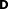, where for pairs of symbols at data subcarrierand $j+1$ the SFBC mapped data symbol matrix, ${\mathbf{D}}_{\left(j\right)}$, follows the next expression.

(16)

In an OFDM system, the signal received at the destination is $\mathbf{y}=\left(\mathbf{s}+\mathbf{p}\right)\mathbf{h}+\mathbf{n}$, where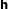is a vector representing the diagonal of the channel matrix and $\mathbf{n}$ represents the additive Gaussian noise. In our $M×L×1$ cooperative system, during phase II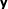follows (2) andis replaced by ${\mathbf{H}}_{\mathsf{\text{ru}}}=\left[{\mathbf{h}}_{\mathsf{\text{ru1}}}{\mathbf{h}}_{\mathsf{\text{ru2}}}\right]$, where ${\mathbf{h}}_{\mathsf{\text{ru1}}}$ and ${\mathbf{h}}_{\mathsf{\text{ru2}}}$ are the diagonals of the $K×K$ matrices that represent the channel frequency responses (CFRs) of the channels between RN and UT.

According to Equations (2)-(4), the extra sources of distortion imply that the accuracy of the initial estimates present some penalties relatively to the case of a point-to-point link. Therefore, in order to improve their accuracies a data-based LS estimation is carried out using the virtual pilots, i.e. the regenerated data symbols $\stackrel{^}{d}$.

As SFBC is used at the RN, the LS estimation based on the data requires a matrix inversion. Considering that two data symbols are encoded in subcarriersand $j+1$, the LS estimate for the equivalent channels is given by

(17)

where ${\stackrel{^}{\mathbf{H}}}_{\mathsf{\text{eq,}}\left(j\right)}^{\mathsf{\text{LS}}}={\left[\begin{array}{cc}\hfill {\stackrel{^}{\mathbf{h}}}_{\mathsf{\text{eq1,}}\left(j\right)}\hfill & \hfill {\stackrel{^}{\mathbf{h}}}_{\mathsf{\text{eq2,}}\left(j\right)}\hfill \end{array}\right]}^{T}$, and ${}_{\mathbf{y}}^{\mathsf{\text{ru,}}\left(j\right)}$ follows (2).

It is important to note that although we have two subcarriers, we obtain a single estimate for each antenna, i.e. if there was no noise, we would obtain the average of the equivalent channels in subcarriersand $j+1$.

The MSE of the estimates in (17) is

$\mathsf{\text{E}}\left\{{\left|e\right|}^{2}\right\}=\frac{1}{J}\left(\sum _{j\in \mathcal{S}}\mathsf{\text{E}}\left\{{\left|{}_{h}^{\left(j\right)}-{}_{ĥ}^{\left(j\right)}\right|}^{2}\right\}\right),$
(18)

whereis the size of the data subcarriers set.

For QPSK with unit power, we derive in Appendix an approximate relation between the error probability ${P}_{\mathsf{\text{e}}}$ and the MSE of SISO and MISO channel estimates. Under the assumption that the correlation involving the data and noise are negligible we have

(19)

where SNR is the signal-to-noise (SNR) ratio assuming that the noise power per subcarrier is ${\sigma }_{n}^{2}$ and the average received signal power (including pilots) is normalised to 1, i.e. $\mathsf{\text{SNR}}=1/{\sigma }_{n}^{2}$. Equation (19) shows that even for a moderate probability of symbol error (e.g. $0.01$) the increase is quite small. Therefore, we can anticipate that even with first data iteration being very inaccurate still there is potential for improving the channel estimates using data.

Moreover, in (17) we consider that the data subcarriers used in the SFBC coding are adjacent. In fact, when designing the transmitted frame, we insert pilots and therefore not all pairs of subcarriers corresponding to one SFBC codeword will be adjacent. For example, if we consider a pilot spacing of 4, i.e. ${N}_{f}=4$, there will be pilots at subcarriers $0,4,8,\dots ,$ and the first SFBC codeword will be transported at the adjacent subcarriers 1 and 2, but the second codeword will be transported at the carriers 3 and 5. In order to overcome that, after performing the LS estimation, we set groups of virtual pilots uniformly spaced. This result in ${N}_{f}-1$ groups of LS estimates with virtual pilots equispaced of ${N}_{f}-1$ as well.

(20)

The pilot-based and the data-based CIRs estimates are combined according to the next expression. An averaging factor guarantees that the resulting power is normalised to 1 and by design this factor results in ${N}_{f}$. After combining the CIRs, the MMSE filtering is performed to enhance the estimate.

(21)

### 3.4. Complexity analysis of the data-aided estimation

The computational complexity of the data-aided iteration is related to the SFBC-decoding and the LS estimation. The merge of both operations requires $5J+{\mathsf{\text{log}}}_{2}\left(J\right)$ multiplications and $2J+J{\mathsf{\text{log}}}_{2}\left(J\right)$ additions per OFDM symbol whereas, according to , the pilot-based iteration requires $L+\left(K{\mathsf{\text{log}}}_{2}\left(K\right)\right)/2$ multiplications and $L{N}_{f}+K{\mathsf{\text{log}}}_{2}\left(K\right)$ additions per OFDM symbol, as well. By analysing only the number of multiplications we found that, despite the effective gains in terms of MSE performance or spectral efficiency, the complexity of the data-aided estimator is about twice of the pilot-based scheme.

## 4. Simulation results

### 4.1. Simulation parameters

In order to evaluate the performance of the presented channel estimation method, we considered the scenario described in Section 2.2 and in the simulation we used the ITU pedestrian channel models A and B  at a speed of . The number of subcarriersset to 1,024 and modulation is QPSK. The transmitted OFDM symbol carried pilot and data subcarriers with a pilot separation ${N}_{f}$. We used the same pilot pattern at the BS and RN and since they were double antenna arrays we allocated different set of pilot subcarriers to perform the estimation. Hence for both the BS and RN, the pilot subcarriers were spaced by $2{N}_{f}$ for each antenna. Since BS and RN are both equipped with an antenna array the resulting MSE of the direct channels B → U (DL) and the relay channels R → U (RL) are obtained by averaging the individuals MSEs.

The simulations were performed assuming uncorrelated antenna channels, the receiver was perfectly synchronised and the insertion of a long enough cyclic prefix in the transmitter ensured that the orthogonality of the subcarriers is maintained after transmission.

We evaluate the estimator performance in three scenarios which are referred in Table 2 as #1, all links have the same statistics; # 2, the links B → R are 10 dB better than the links B → U and R → U; # 3, the overall relay links are 10 dB better than the direct ones. The results are presented in terms of MSE per ${E}_{\mathsf{\text{b}}}/{N}_{0}$ of the direct link.

### 4.2. MSE channel estimation performance

Figure 10 shows the MSE of the CFR estimate of the relay and the direct links, employing the pilot and the pilot-data estimators, considering the Scenario #1 and considering the channel ITU Pedestrian model A. It shows that the pilot-based estimates of the RL present a penalty over the DL that accounts for the extra source of noise aforementioned. It also shows that the pilot-data-based estimation method can significantly overcome such penalisation and provides a performance better than the DL for all ${N}_{f}$ considered. For high values of ${E}_{\mathsf{\text{b}}}/{N}_{0}$ as ${N}_{f}$ increases the relative gain provided by the data-aided estimator increases as well. From the figure, we verify that for , the pilot-data-based results provide 5 and 3 dB gain over the estimator using only pilots for values 16 and 4 of ${N}_{f}$, respectively. For low values of ${E}_{\mathsf{\text{b}}}/{N}_{0}$ the gain is smaller but even for ${E}_{\mathsf{\text{b}}}/{N}_{0}$ as low as 0 dB we still gain 2 dB over the pilot-based estimator when ${N}_{f}=4$. The gain reduction as ${E}_{\mathsf{\text{b}}}/{N}_{0}$ decreases is understandable since the probability of error in the first iteration increases and therefore several virtual pilots used for the second iteration are erroneous. Moreover, inspection of the curves of Figure 10 shows us that the MSE of the pilot-data-based estimator for a given ${N}_{f}$ is always below the one achieved considering the pilot-based estimator with pilot separation of ${N}_{f}/2$. This means that the total number of pilots can be halved leading to an improved spectral efficiency. In Figure 10, we also present, in green line, the performance of the pilot-data estimator for ${N}_{f}=4$ when perfect decoded data are used instead of regenerated data. Considering several iterations in this algorithm, the gain expected would be smaller than 0.77 dB, which is the difference in performance of the pilot-data-based estimator when perfect and regenerated data, green and black lines, respectively, are employed. This difference is smaller, 0.4 dB, considering the ITU pedestrian channel model B, as presented in next results. According to our results, with only one data iteration, the proposed estimator provides significant gains over the pilot-based estimator, black and red results.

In Figure 11, we present the same type of results but considering for the channel Pedestrian model B. This channel has much lower coherence bandwidth than model A and we can observe that with ${N}_{f}=16$ the pilot-data-based estimator starts presenting an error floor for high values of ${E}_{\mathsf{\text{b}}}/{N}_{0}$. This was explained in Section 3.2, because Alamouti coding we obtain in fact the average channel of two subcarriers. With model A, the channels for two adjacent subcarriers are strongly correlated and averaging introduces no noticeable error, but for model B the correlation is lower than model A and averaging effect starts to be noticeable for high values of ${E}_{\mathsf{\text{b}}}/{N}_{0}$. This error floor effect occurs for all the values of ${N}_{f}$ but the larger the pilot separation the faster (in terms of ${E}_{\mathsf{\text{b}}}/{N}_{0}$) it starts to be noticeable. This effect can be reduced by using different weights for the data and pilot contributions in (21). Also it is worthwhile emphasise that in scenarios with highly frequency selective channels, the use of specific techniques such as the ones presented in  can mitigate the Alamouti decoding error and therefore improve the estimator performance. In Figure 11, we also present, in green line, the performance of the pilot-data estimator for ${N}_{f}=4$ when perfect decoded data are used instead of regenerated one.

Figures 12, 13, 14 and 15 present the estimators MSE performance considering the Scenarios # 2 and # 3. The choice of these scenarios for downlink derives from the fact that, in most real situations, the cooperative links have higher transmission quality conditions than the direct link. The results presented in Figures 12, 13, 14 and 15 emphasise the benefits of cooperation in terms of MSE and the improvements that are achieved using the proposed pilot-data scheme as well.

Figures 12 and 13 present the results relative to Scenario # 2, for channel models A and B, respectively. In both cases, the pilot-based estimates of the RL and DL present approximately the same performance. This is due to the fact that in the case that the links between BS and RNs are highly reliable, most of the data information is successfully detected at the RN, which has a positive impact on the relays links. We can observe that the proposed pilot-data estimator for ${N}_{f}=16$ achieves approximately the same performance of the pilot-based one for ${N}_{f}=4$; therefore, requiring only 1/4 of the pilot subcarriers used by the pilot-based method.

Figures 14 and 15 present the results relative to Scenario # 3 for channel models A and B, respectively. These results show that in such scenario both links B → R and R → U have higher quality conditions over the direct one. In this case, the noise variances have a minor effect on the pilot-based estimates and due that the RL performance overreaches the DL one. The proposed scheme nevertheless can improve the RL performance. For ${N}_{f}=8$, the proposed estimator presents a performance close to the pilot-data performance considering only 1/2 of the pilots used by the pilot-based estimator, i.e. ${N}_{f}=4$. In this scenario, the MSE of the pilot-data-based estimator for a given ${N}_{f}$ is quite close to the one achieved considering the pilot-based estimator with pilot separation of ${N}_{f}/2$.

## 5. Conclusion

We proposed a pilot-data-based estimation algorithm for an OFDM-based cooperative scenario where spatial diversity provided by SFBC is complemented with the use of a half-duplex RN using the EF protocol. The proposed method consists of two iterations and uses the MMSE criterion to design the estimator for both the pilot-based and data-aided iterations. The data-aided estimation component is carried out using the regenerated data symbols as virtual pilots. In different scenarios, the results have shown that for the same pilot density the MSE is reduced approximately by 3 dB or alternatively requires half of pilot density to achieve the same performance therefore improving the overall system spectral efficiency with only one data iteration.

It is clear from the presented results that the proposed pilot-data-based method has significant interest for application in next generation wireless networks for which cooperation is anticipated.

### Appendix

Throughout this section, we use the following definitions:

• The received power is given by

• The power at the pilot subcarriers is

• The noise variance per subcarriers is represented by ${\sigma }_{n}^{2}$ and therefore the total power is given by .

• If there is any distinction among pilot and data subcarriers the SNR is

$\mathsf{\text{SNR}}=\frac{{\sigma }_{j}^{2}\mathsf{\text{E}}\left\{\sum _{k}{\left|{h}_{\left(k\right)}\right|}^{2}\right\}}{K{\sigma }_{n}^{2}}.$
• If ${\sigma }_{j}^{2}=1,\mathsf{\text{E}}\left\{{\left|{h}_{\left(k\right)}\right|}^{2}\right\}=1$ and $\mathsf{\text{E}}\left\{\sum _{k}{\left|{h}_{\left(k\right)}\right|}^{2}\right\}=K$ the SNR is given by .

### SISO channel

According to the LS estimation in a SISO channel, the error in the channel estimates is

$e={h}_{\left(k\right)}-{ĥ}_{\left(k\right)}={h}_{\left(k\right)}\left(1-{d}_{\left(k\right)}{\stackrel{^}{d}}_{\left(k\right)}^{*}\right)+{w}_{\left(k\right)}{\stackrel{^}{d}}_{\left(k\right)}^{*},$
(22)

where ${d}_{\left(k\right)}$ and ${\stackrel{^}{d}}_{\left(k\right)}$ are the transmitted and the regenerated data, respectively, and for QPSK ${\left|{d}_{\left(k\right)}\right|}^{2}=1$ and ${d}_{\left(k\right)}^{*}=1/{d}_{\left(k\right)}$.

The squared norm of the error vector is given by

$\begin{array}{c}{\left|e\right|}^{2}={\left|{h}_{\left(k\right)}\right|}^{2}{\left|1-{d}_{\left(k\right)}{\stackrel{^}{d}}_{\left(k\right)}^{*}\right|}^{2}+{\left|{w}_{\left(k\right)}\right|}^{2}+2\left|{h}_{\left(k\right)}\right|\left|1-{d}_{\left(k\right)}{\stackrel{^}{d}}_{\left(k\right)}^{*}\right|\left|{w}_{\left(k\right)}{\stackrel{^}{d}}_{\left(k\right)}^{*}\right|\\ E\left\{{\left|e\right|}^{2}\right\}=E\left\{{\left|{h}_{\left(k\right)}\right|}^{2}\right\}E\left\{{\left|1-{d}_{\left(k\right)}{\stackrel{^}{d}}_{\left(k\right)}^{*}\right|}^{2}\right\}+{\sigma }_{n}^{2}+E\left\{2\left|{h}_{\left(k\right)}\right|\left|1-{d}_{\left(k\right)}{\stackrel{^}{d}}_{\left(k\right)}^{*}\right|\left|{w}_{\left(k\right)}{\stackrel{^}{d}}_{\left(k\right)}^{*}\right|\right\}.\end{array}$
(23)

Since that ${\left|1-{d}_{\left(k\right)}{\stackrel{^}{d}}_{\left(k\right)}^{*}\right|}^{2}={\left|1-{d}_{\left(k\right)}/{}_{\stackrel{^}{d}}^{\left(k\right)}\right|}^{2}={\left|{}_{\stackrel{^}{d}}^{\left(k\right)}-{d}_{\left(k\right)}\right|}^{2}/{\left|{}_{\stackrel{^}{d}}^{\left(k\right)}\right|}^{2}={\left|\epsilon \right|}^{2}$. For QPSK ${d}_{\left(k\right)}=\left(1+j\right)/\sqrt{2}$ and therefore ${}_{\stackrel{^}{d}}^{\left(k\right)}$ follows

According to the table above the expected value of the error is given by

$\begin{array}{c}\phantom{\rule{1em}{0ex}}\phantom{\rule{1em}{0ex}}\phantom{\rule{1em}{0ex}}\phantom{\rule{1em}{0ex}}\mathsf{\text{E}}\left\{{\left|e\right|}^{2}\right\}=\mathsf{\text{E}}\left\{{\left|{h}_{\left(k\right)}\right|}^{2}\right\}\mathsf{\text{E}}\left\{{\left|\epsilon \right|}^{2}\right\}+{\sigma }_{n}^{2}\\ \mathsf{\text{E}}\left\{{\left|e\right|}^{2}\right\}=\mathsf{\text{E}}\left\{{\left|{h}_{\left(k\right)}\right|}^{2}\right\}\left(2{P}_{\mathsf{\text{e}}}/2+2{P}_{\mathsf{\text{e}}}/2\right)+{\sigma }_{n}^{2}=2\mathsf{\text{E}}\left\{{\left|{h}_{\left(k\right)}\right|}^{2}\right\}{P}_{\mathsf{\text{e}}}+{\sigma }_{n}^{2}\end{array}$
(24)

Since we assume $\mathsf{\text{E}}\left\{{\left|{h}_{\left(k\right)}\right|}^{2}\right\}=1$ and

$\begin{array}{c}\mathsf{\text{E}}\left\{{\left|e\right|}^{2}\right\}\approx 2{P}_{\mathsf{\text{e}}}+{\sigma }_{n}^{2}\\ \mathsf{\text{E}}\left\{{\left|e\right|}^{2}\right\}\approx {\sigma }_{n}^{2}\left(1+2{P}_{\mathsf{\text{e}}}\mathsf{\text{SNR}}\right).\end{array}$
(25)

### MISO channel

Since our scenario is a cooperative $2×2×1$ and the LS data estimation is used to estimate the channels R → U which is $2×1$, we need provide expression for the squared norm of the error in this case as well.

In a MISO system, the signal to be hard-decoded at the destination is given by

$\left\{\begin{array}{l}{}_{\stackrel{^}{d}}^{\left(k\right)}={g}_{1}^{*}{}_{y}^{\left(k\right)}+{}_{g}^{2}{y}_{\left(k+1\right)}^{*},{g}_{1}={h}_{\mathsf{\text{1,}}\left(k\right)}/\left(\sqrt{2}{\sigma }_{n}^{2}\right)\\ {}_{\stackrel{^}{d}}^{\left(k+1\right)}=-{}_{g}^{2}{y}_{\left(k\right)}^{*}+{g}_{1}^{*}{}_{y}^{\left(k+1\right)},{g}_{2}={h}_{\mathsf{\text{2,}}\left(k\right)}/\left(\sqrt{2}{\sigma }_{n}^{2}\right)\end{array}\right\$
(26)

where are the channels per subcarriersbetween the transmitter and the receiver and the received signal ${y}_{\left(k\right)}$ is given by (2) and ${g}_{1,2}$ are the equalisation coefficients.

$\left\{\begin{array}{c}{}_{y}^{\left(k\right)}=\frac{1}{\sqrt{2}}\left({h}_{1,\left(k\right)}{}_{\stackrel{^}{d}}^{\left(k\right)}-{h}_{2\left(k+1\right)}{\stackrel{^}{d}}_{\left(k+1\right)}^{*}\right)+{}_{n}^{\left(k\right)}\\ {}_{y}^{\left(k+1\right)}=\frac{1}{\sqrt{2}}\left({h}_{\mathsf{\text{2}},\left(k\right)}{\stackrel{^}{d}}_{\left(k\right)}^{*}+{h}_{1}{}_{\stackrel{^}{d}}^{\left(k+1\right)}\right)+{}_{n}^{\left(k+1\right)}\end{array}\right\,$
(27)

The LS estimation in a MISO system is given by

$\begin{array}{c}\mathbf{H}={\mathbf{D}}^{-1}\mathbf{Y},\mathbf{D}=\sqrt{2}\left(\begin{array}{cc}\hfill {}_{\stackrel{^}{d}}^{\left(k\right)}\hfill & \hfill -{\stackrel{^}{d}}_{\left(k+1\right)}^{*}\hfill \\ \hfill {}_{\stackrel{^}{d}}^{\left(k+1\right)}\hfill & \hfill {\stackrel{^}{d}}_{\left(k\right)}^{*}\hfill \end{array}\right)⇒{\mathbf{D}}^{-1}=\frac{\sqrt{2}}{{}_{\stackrel{^}{d}}^{\left(k\right)}{\stackrel{^}{d}}_{\left(k\right)}^{*}+{}_{\stackrel{^}{d}}^{\left(k+1\right)}{\stackrel{^}{d}}_{\left(k+1\right)}^{*}}\left(\begin{array}{cc}\hfill {}_{\stackrel{^}{d}}^{\left(k\right)}\hfill & \hfill -{\stackrel{^}{d}}_{\left(k+1\right)}^{*}\hfill \\ \hfill {}_{\stackrel{^}{d}}^{\left(k+1\right)}\hfill & \hfill {\stackrel{^}{d}}_{\left(k\right)}^{*}\hfill \end{array}\right)\\ \phantom{\rule{1em}{0ex}}\phantom{\rule{1em}{0ex}}\phantom{\rule{1em}{0ex}}\phantom{\rule{1em}{0ex}}\left\{\begin{array}{l}{}_{ĥ}^{1\left(k\right)}=\frac{\sqrt{2}}{{}_{\stackrel{^}{d}}^{\left(k\right)}{\stackrel{^}{d}}_{\left(k\right)}^{*}+{}_{\stackrel{^}{d}}^{\left(k+1\right)}{\stackrel{^}{d}}_{\left(k+1\right)}^{*}}\left({\stackrel{^}{d}}_{\left(k\right)}^{*}{}_{y}^{\left(k\right)}+{\stackrel{^}{d}}_{\left(k+1\right)}^{*}{}_{y}^{\left(k+1\right)}\right)+{}_{n}^{\left(k\right)}\\ {}_{ĥ}^{2\left(k\right)}=\frac{\sqrt{2}}{{}_{\stackrel{^}{d}}^{\left(k\right)}{\stackrel{^}{d}}_{\left(k\right)}^{*}+{}_{\stackrel{^}{d}}^{\left(k+1\right)}{\stackrel{^}{d}}_{\left(k+1\right)}^{*}}\left(-{}_{\stackrel{^}{d}}^{\left(k+1\right)}{}_{y}^{\left(k\right)}+{}_{\stackrel{^}{d}}^{\left(k\right)}{}_{y}^{\left(k+1\right)}\right)+{}_{n}^{\left(k+1\right)}\end{array}\right\\end{array}$
(28)
$\left\{\begin{array}{c}{}_{ĥ}^{1\left(k\right)}=\frac{{}_{h}^{1\left(k\right)}\left({\stackrel{^}{d}}_{\left(k\right)}^{*}{}_{d}^{\left(k\right)}+{\stackrel{^}{d}}_{\left(k+1\right)}^{*}{}_{d}^{\left(k+1\right)}\right)}{{}_{\stackrel{^}{d}}^{\left(k\right)}{\stackrel{^}{d}}_{\left(k\right)}^{*}+{}_{\stackrel{^}{d}}^{\left(k+1\right)}{\stackrel{^}{d}}_{\left(k+1\right)}^{*}}+\frac{{\stackrel{^}{d}}_{\left(k\right)}^{*}{}_{n}^{\left(k\right)}}{{}_{\stackrel{^}{d}}^{\left(k\right)}{\stackrel{^}{d}}_{\left(k\right)}^{*}+{}_{\stackrel{^}{d}}^{\left(k+1\right)}{\stackrel{^}{d}}_{\left(k+1\right)}^{*}}+\frac{{\stackrel{^}{d}}_{\left(k+1\right)}^{*}{}_{n}^{\left(k+1\right)}}{{}_{\stackrel{^}{d}}^{\left(k\right)}{\stackrel{^}{d}}_{\left(k\right)}^{*}+{}_{\stackrel{^}{d}}^{\left(k+1\right)}{\stackrel{^}{d}}_{\left(k+1\right)}^{*}}\\ {}_{ĥ}^{2\left(k\right)}=\frac{{}_{h}^{2\left(k\right)}\left({d}_{\left(k\right)}^{*}{}_{\stackrel{^}{d}}^{\left(k\right)}+{d}_{\left(k+1\right)}^{*}{}_{\stackrel{^}{d}}^{\left(k+1\right)}\right)}{{}_{\stackrel{^}{d}}^{\left(k\right)}{\stackrel{^}{d}}_{\left(k\right)}^{*}+{}_{\stackrel{^}{d}}^{\left(k+1\right)}{\stackrel{^}{d}}_{\left(k+1\right)}^{*}}+\frac{{}_{\stackrel{^}{d}}^{\left(k\right)}{}_{n}^{\left(k+1\right)}}{{}_{\stackrel{^}{d}}^{\left(k\right)}{\stackrel{^}{d}}_{\left(k\right)}^{*}+{}_{\stackrel{^}{d}}^{\left(k+1\right)}{\stackrel{^}{d}}_{\left(k+1\right)}^{*}}+\frac{-{}_{\stackrel{^}{d}}^{\left(k+1\right)}{}_{n}^{\left(k\right)}}{{}_{\stackrel{^}{d}}^{\left(k\right)}{\stackrel{^}{d}}_{\left(k\right)}^{*}+{}_{\stackrel{^}{d}}^{\left(k+1\right)}{\stackrel{^}{d}}_{\left(k+1\right)}^{*}}\end{array}\right\$
(29)

The error in (4) is given by the following expression:

$\left\{\begin{array}{c}{e}_{1}={}_{h}^{1\left(k\right)}-{}_{ĥ}^{1\left(k\right)}\\ {e}_{2}={}_{h}^{2\left(k\right)}-{}_{ĥ}^{2\left(k\right)}\end{array}\right\.$
(30)

By replacing (8) in (9) we may obtain

$\left\{\begin{array}{c}{e}_{1}={}_{h}^{1\left(k\right)}\left(1-\frac{\left({\stackrel{^}{d}}_{\left(k\right)}^{*}{}_{d}^{\left(k\right)}+{\stackrel{^}{d}}_{\left(k+1\right)}^{*}{}_{d}^{\left(k+1\right)}\right)}{{}_{\stackrel{^}{d}}^{\left(k\right)}{\stackrel{^}{d}}_{\left(k\right)}^{*}+{}_{\stackrel{^}{d}}^{\left(k+1\right)}{\stackrel{^}{d}}_{\left(k+1\right)}^{*}}\right)+\frac{1}{{}_{\stackrel{^}{d}}^{\left(k\right)}{\stackrel{^}{d}}_{\left(k\right)}^{*}+{}_{\stackrel{^}{d}}^{\left(k+1\right)}{\stackrel{^}{d}}_{\left(k+1\right)}^{*}}\left({\stackrel{^}{d}}_{\left(k\right)}^{*}{}_{n}^{\left(k\right)}+{\stackrel{^}{d}}_{\left(k+1\right)}^{*}{}_{n}^{\left(k+1\right)}\right)\\ {e}_{2}={}_{h}^{2\left(k\right)}\left(1-\frac{\left({d}_{\left(k\right)}^{*}{}_{\stackrel{^}{d}}^{\left(k\right)}+{d}_{\left(k+1\right)}^{*}{}_{\stackrel{^}{d}}^{\left(k+1\right)}\right)}{{}_{\stackrel{^}{d}}^{\left(k\right)}{\stackrel{^}{d}}_{\left(k\right)}^{*}+{}_{\stackrel{^}{d}}^{\left(k+1\right)}{\stackrel{^}{d}}_{\left(k+1\right)}^{*}}\right)+\frac{1}{{}_{\stackrel{^}{d}}^{\left(k\right)}{\stackrel{^}{d}}_{\left(k\right)}^{*}+{}_{\stackrel{^}{d}}^{\left(k+1\right)}{\stackrel{^}{d}}_{\left(k+1\right)}^{*}}\left({}_{\stackrel{^}{d}}^{\left(k\right)}{}_{n}^{\left(k+1\right)}-{}_{\stackrel{^}{d}}^{\left(k+1\right)}{}_{n}^{\left(k\right)}\right)\end{array}\right\.$
(31)

The error vector is given by:

$\left\{\begin{array}{l}\left|{e}_{1}\right|=\frac{1}{\left|x\right|}\left[\left|{}_{h}^{1\left(k\right)}\right|\left|x-\left({\stackrel{^}{d}}_{\left(k\right)}^{*}{}_{d}^{\left(k\right)}+{\stackrel{^}{d}}_{\left(k+1\right)}^{*}{}_{d}^{\left(k+1\right)}\right)\right|+\left|{\stackrel{^}{d}}_{\left(k\right)}^{*}{}_{n}^{\left(k\right)}\right|+\left|{\stackrel{^}{d}}_{\left(k+1\right)}^{*}{}_{n}^{\left(k+1\right)}\right|\right]\\ \left|{e}_{2}\right|=\frac{1}{\left|x\right|}\left[\left|{}_{h}^{2\left(k\right)}\right|\left|x-\left({d}_{\left(k\right)}^{*}{}_{\stackrel{^}{d}}^{\left(k\right)}+{d}_{\left(k+1\right)}^{*}{}_{\stackrel{^}{d}}^{\left(k+1\right)}\right)\right|+\left|{}_{\stackrel{^}{d}}^{\left(k\right)}{}_{n}^{\left(k+1\right)}\right|+\left|-{}_{\stackrel{^}{d}}^{\left(k+1\right)}{}_{n}^{\left(k\right)}\right|\right]\end{array}\right\,x={}_{\stackrel{^}{d}}^{\left(k\right)}{\stackrel{^}{d}}_{\left(k\right)}^{*}+{}_{\stackrel{^}{d}}^{\left(k+1\right)}{\stackrel{^}{d}}_{\left(k+1\right)}^{*}.$
(32)

We assume that ${d}_{\left(k\right)}{d}_{\left(k\right)}^{*}=\left|{d}_{\left(k\right)}\right|$ and for QPSK $\left|{d}_{\left(k\right)}\right|=1$, therefore $x=2$ and the expected value of the squared error is

$\left\{\begin{array}{c}\mathsf{\text{E}}\left\{{\left|{e}_{1}\right|}^{2}\right\}=\frac{1}{4}\left[\mathsf{\text{E}}\left\{{\left|{}_{h}^{1\left(k\right)}\right|}^{2}\right\}\mathsf{\text{E}}\left\{{\left|2-\left({\stackrel{^}{d}}_{\left(k\right)}^{*}{}_{d}^{\left(k\right)}+{\stackrel{^}{d}}_{\left(k+1\right)}^{*}{}_{d}^{\left(k+1\right)}\right)\right|}^{2}\right\}+{\sigma }_{n,\left(k\right)}^{2}+{\sigma }_{n,\left(k+1\right)}^{2}\right]\\ \mathsf{\text{E}}\left\{{\left|{e}_{2}\right|}^{2}\right\}=\frac{1}{4}\left[\mathsf{\text{E}}\left\{{\left|{}_{h}^{2\left(k\right)}\right|}^{2}\right\}\mathsf{\text{E}}\left\{{\left|2-\left({d}_{\left(k\right)}^{*}{}_{\stackrel{^}{d}}^{\left(k\right)}+{d}_{\left(k+1\right)}^{*}{}_{\stackrel{^}{d}}^{\left(k+1\right)}\right)\right|}^{2}\right\}+{\sigma }_{n,\left(k+1\right)}^{2}+{\sigma }_{n,\left(k\right)}^{2}\right]\end{array}\right\.$
(33)

Since that ${\left|2-\left({\stackrel{^}{d}}_{\left(k\right)}^{*}{}_{d}^{\left(k\right)}+{\stackrel{^}{d}}_{\left(k+1\right)}^{*}{}_{d}^{\left(k+1\right)}\right)\right|}^{2}={\left|2-\left({}_{d}^{\left(k\right)}/{}_{\stackrel{^}{d}}^{\left(k\right)}+{}_{d}^{\left(k+1\right)}/{}_{\stackrel{^}{d}}^{\left(k+1\right)}\right)\right|}^{2}={\left|\epsilon \right|}^{2}$. For QPSK ${d}_{\left(k\right)}=\left(1+j\right)/\sqrt{2}$ and therefore

According to the table above, the expected value of the error square is given by

$\begin{array}{c}\phantom{\rule{1em}{0ex}}\phantom{\rule{1em}{0ex}}\phantom{\rule{1em}{0ex}}\phantom{\rule{1em}{0ex}}\mathsf{\text{E}}\left\{{\left|{e}_{1}\right|}^{2}\right\}=\frac{1}{4}\mathsf{\text{E}}\left\{{\left|{h}_{1,\left(k\right)}\right|}^{2}\right\}\mathsf{\text{E}}\left\{{\left|\epsilon \right|}^{2}\right\}+2{\sigma }_{n}^{2}\\ \mathsf{\text{E}}\left\{{\left|{e}_{1}\right|}^{2}\right\}=\frac{1}{4}\mathsf{\text{E}}\left\{{\left|{h}_{1,\left(k\right)}\right|}^{2}\right\}\left(2{P}_{\mathsf{\text{e}}}/4+2{P}_{\mathsf{\text{e}}}/4+2{P}_{\mathsf{\text{e}}}/4+2{P}_{\mathsf{\text{e}}}/4\right)+2{\sigma }_{n}^{2}.\end{array}$
(34)

Since we assume $\mathsf{\text{E}}\left\{{\left|{h}_{\left(k\right)}\right|}^{2}\right\}=1$ and

$\begin{array}{c}\mathsf{\text{E}}\left\{{\left|{e}_{1}\right|}^{2}\right\}\approx \frac{1}{4}\mathsf{\text{E}}\left\{{\left|{h}_{1,\left(k\right)}\right|}^{2}\right\}2{P}_{\mathsf{\text{e}}}+2{\sigma }_{n}^{2}\\ \mathsf{\text{E}}\left\{{\left|{e}_{1}\right|}^{2}\right\}\approx \frac{1}{2}{\sigma }_{n}^{2}\left(1+{P}_{\mathsf{\text{e}}}\mathsf{\text{SNR}}\right).\end{array}$
(35)

## References

1. Liu KJR, Sadek AK, Su W, Kwasinski A: Cooperative Communications and Networking. Cambridge University Press, New York; 2008.

2. Laneman JN, Tse DNC, Wornell GW: Cooperative diversity in wireless networks: efficient protocols and outage behaviour. IEEE Trans Inf Theory 2004, 50(12):3062-3080. 10.1109/TIT.2004.838089

3. Teodoro S, Silva A, Gil JM, Gameiro A: Virtual MIMO schemes for downlink space-frequency coding OFDM systems. IEEE International Symposium on Personal, Indoor and Mobile Radio Communication, 2009. PIMRC'09, Tokyo 2009, 1322-1326.

4. Moço A, Teodoro S, Silva A, Gameiro A: Single and multiple antenna relay-assisted techniques for uplink and downlink OFDM systems. Int J Adv Syst Meas 2010, 3(2):22-34.

5. Muhaidat H, Uysal M, Adve R: Pilot-symbol-assisted detection scheme for distributed orthogonal space-time block coding. IEEE Trans Wirel Commun 2009, 3(3):1057-1061.

6. Gedik B, Uysal M: Impact of imperfect channel estimation on the performance of amplify-and-forward relaying. IEEE Trans Wirel Commun 2009, 8(3):1468-1479.

7. Ikki SS, Feteiha M, Uysal M: Performance analysis of cooperative diversity networks with imperfect channel estimation over Rician fading channels. IEEE International Conference on Communications, 2009. ICC'09, Cape Town 2010, 160-165.

8. Han S, Ahn S, Oh E, Hong D: Effect of channel-estimation error on BER performance in cooperative transmission. IEEE Trans Veh Technol 2009, 58(4):2083-2088.

9. Amin O, Ikki SS, Uysal M: On the performance analysis of multiple-relay cooperative diversity systems with channel estimation errors. IEEE Trans Veh Technol 2011, 60(5):2050-2059.

10. Choi J: MMSE-based distributed beamforming in cooperative relay networks. IEEE Trans Commun 2011, 59(5):1346-1356.

11. Neves D, Ribeiro C, Silva A, Gameiro A: Channel estimation schemes for OFDM relay-assisted systems. IEEE Vehicular Technology Conference Spring, 2009.VTC'09-SPRING, Barcelona 2009, 1-5.

12. Ma J, Orlik P, Zhang J, Li GY: Pilot matrix design for estimating cascaded channels in two-hop MIMO amplify-and-forward relay systems. IEEE Trans Wirel Commun 2011, 10(6):1946-1965.

13. Wang T, de Lamare RC, Mitchell PD: BEACON channel estimation for cooperative wireless sensor networks based on data selection. IEEE International Symposium on Wireless Systems, 2010. ISWS'10, York 2010, 140-144.

14. Wang T, de Lamare RC, Mitchell PD: Low-complexity set-membership channel estimation for cooperative wireless sensor networks. IEEE Trans Veh Technol 2011, 60(6):2594-2607.

15. Tu J, Cai Y: Time-domain channel estimation for amplify-and-forward relay in OFDM systems. IEEE International Conference on Wireless Communication and Signal Processing, 2010. ICWCSP'10, Suzhou 2010, 1-5.

16. Roemer F, Haardt M: Tensor-based channel estimation and iterative refinements for two-way relaying with multiple antennas and spatial reuse. IEEE Trans Signal Process 2010, 58(11):5720-5735.

17. Xing C, Ma S, Wu YC, Ng TS: Transceiver design for dual-hop non-regenerative MIMO-OFDM relay systems under channel uncertainties. IEEE Trans Signal Process 2011, 58(12):6325-6339.

18. Wang G, Gao F, Tellambura C: Superimposed pilots aided joint CFO and channel estimation for ZP-OFDM modulated two-way. IEEE Vehicular Technology Conference Fall, 2010. VTC'10-FALL, Ottawa 2010, 1-5.

19. Pang J, Shen G, Wang D, Jiang L, Wang W: Channel estimation and optimal training design for amplify and forward MIMO relay channel under spatial fading correlation. IEEE Vehicular Technology Conference Fall, 2010. VTC'10-FALL, Ottawa 2010, 1-5.

20. Song M, Kim D, Im G: Recursive channel estimation method for OFDM-BASED cooperative systems. IEEE Commun Lett 2010, 14(11):1029-1031.

21. Aerts N, Avram I, Duyck D, Moeneclaey M: EM based channel estimation in an amplify-and-forward relaying network. IEEE Proceeding on Personal Indoor and Mobile Radio Communications, 2010. PIRMC'10, Istanbul 2010, 724-729.

22. Sheu JS, Sheen WH: An EM algorithm-based channel estimation for OFDM amplify-and-forward relaying systems. IEEE International Conference on Communication, 2010. ICC'10, Cape Town 2010, 1-5.

23. Zhou X, Lamahewa TA, Sadeghi P: Kalman filter-based channel estimation for amplify and forward relay communications. IEEE Asilomar Conference Signals Systems and Computers, 2009. ACSSC'09, Pacific Grove 2009, 1498-1502.

24. Neves D, Ribeiro C, Silva A, Gameiro A: A pilot-data based channel estimation method for OFDM relay-assisted systems. IEEE Vehicular Technology Conference Fall, 2011. VTC'11-Fall, San Francisco 2011, 1-5.

25. Neves D, Ribeiro C, Silva A, Gameiro A: A time domain channel estimation scheme for equalize-and-forward relay-assisted systems. IEEE Vehicular Technology Conference Fall, 2010. VTC'10-FALL, Ottawa 2010, 1-5.

26. Ribeiro C, Gameiro A: An OFDM symbol design for reduced complexity MMSE channel estimation. J Commun 2008, 3(4):26-33.

27. 3GPP TS 36.201 V8.1.0: 3rd "Generation Partnership Project", Technical Specification Group Radio Access Network Evolved Universal Terrestrial Radio Access (E-UTRA), LTE Physical Layer - General Description. 2007.

28. Vielmon A, Li Y, Barry J: Performance of Alamouti transmit diversity over time-varying rayleigh-fading channels. IEEE Trans Wirel Commun 2004, 3(55):1369-1373.

## Acknowledgements

The authors acknowledge the support of the European project CODIV--FP7/ICT/2007/215477, the Portuguese projects CADWIN--PTDC/EEA-TEL/099241/2008 and CROWN--PTDC/EEA-TEL/115828/2009, and the Portuguese Foundation for Science and Technology (FCT) grant for D. Neves.

## Author information

Authors

### Corresponding author

Correspondence to Darlene Neves.

### Competing interests

The authors declare that they have no competing interests.

## Authors’ original submitted files for images

Below are the links to the authors’ original submitted files for images.

## Rights and permissions

Reprints and Permissions

Neves, D., Ribeiro, C., Silva, A. et al. An iterative pilot-data-aided estimator for SFBC relay-assisted OFDM-based systems. EURASIP J. Adv. Signal Process. 2012, 74 (2012). https://doi.org/10.1186/1687-6180-2012-74

• Accepted:

• Published:

• DOI: https://doi.org/10.1186/1687-6180-2012-74

### Keywords

• Space Frequency Block Coding (SFBC)
• Channel Estimator
• Equivalent Channel
• Pilot Density
• Pilot-based Estimation